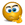# Time difference im very confuse

## Excel Help for Time Difference Im Very Confuse in Analyzing Corporate Financial DataDear Sir  am very confuse sir your formula for sutable only 11.30 to 13 sir, i cant use any other time sir please give some itea Thanks & Best Regards,JONESH KUMAR G joneshkumar g Posted by jonus.venus on 31 Mar 2012Rate this: (3/5 from 1 vote) OK - So we assume here that the decimal part of the time represents the number of minutes.  the solution here is to separate the whole number (hours) and the decimal (minutes) to calculate the number of hours difference. We can do this by using the INT function that returns the whole number and then process the decimal part to calculate the fraction of an hour.Assuming that the start time is in cell A1 and the end time is in B1, the formula to calculate the number of hours difference is:=INT(B1-A1)+((B1-A1)-INT(B1-A1)-0.4)/0.6 https://doodlebaseball.io/ Posted by nuncravat on 14 Nov 2023